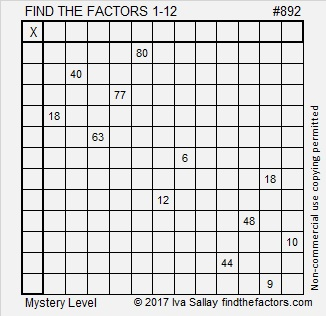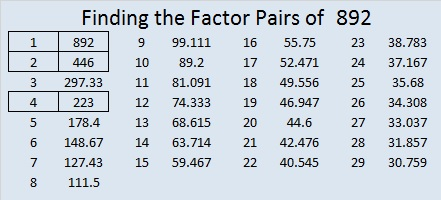# 892 Tribute to The Mysteries of Harris Burdick

When I finished making today’s puzzle, I remembered a particular picture from The Mysteries of Harris Burdick. Can you guess which picture that would be?Print the puzzles or type the solution on this excel file: 12 factors 886-896

This popular children’s book contains only pictures with short captions. Children use their imaginations to write short stories for the curious pictures and captions. The book is available in municipal libraries everywhere and on amazon.com.892 looks interesting in a couple of different bases:

• It is 4044 in BASE 6, because 4(6³) + 4(6) + 4(1) = 4(216 + 6 + 1) = 4(223) = 892
• It is 161 in BASE 27, because 1(27²) + 6(27) + 1(1) = 892

892 is also the sum of consecutive prime numbers: 443 + 449 = 892

• 892 is a composite number.
• Prime factorization: 892 = 2 × 2 × 223, which can be written 892 = 2² × 223
• The exponents in the prime factorization are 2 and 1. Adding one to each and multiplying we get (2 + 1)(1 + 1) = 3 × 2  = 6. Therefore 892 has exactly 6 factors.
• Factors of 892: 1, 2, 4, 223, 446, 892
• Factor pairs: 892 = 1 × 892, 2 × 446, or 4 × 223
• Taking the factor pair with the largest square number factor, we get √892 = (√4)(√223) = 2√223 ≈ 29.866369This site uses Akismet to reduce spam. Learn how your comment data is processed.